# Hungarian Method Examples

Now we will examine a few highly simplified illustrations of Hungarian Method for solving an assignment problem.

Later in the chapter, you will find more practical versions of assignment models like Crew assignment problem, Travelling salesman problem, etc.

Example-1, Example-2

##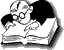Example 1: Hungarian Method

The Funny Toys Company has four men available for work on four separate jobs. Only one man can work on any one job. The cost of assigning each man to each job is given in the following table. The objective is to assign men to jobs in such a way that the total cost of assignment is minimum.

Job
Person 1 2 3 4
A 20 25 22 28
B 15 18 23 17
C 19 17 21 24
D 25 23 24 24

Solution.

This is a minimization example of assignment problem. We will use the Hungarian Algorithm to solve this problem.

####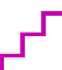Step 1

Identify the minimum element in each row and subtract it from every element of that row. The result is shown in the following table.

"A man has one hundred dollars and you leave him with two dollars, that's subtraction." -Mae West

Table

On small screens, scroll horizontally to view full calculation

Job
Person 1 2 3 4
A 0 5 2 8
B 0 3 8 2
C 2 0 4 7
D 2 0 1 1

Step 2

Identify the minimum element in each column and subtract it from every element of that column.

Table

Job
Person 1 2 3 4
A 0 5 1 7
B 0 3 7 1
C 2 0 3 6
D 2 0 0 0

Step 3

Make the assignments for the reduced matrix obtained from steps 1 and 2 in the following way:

1. For each row or column with a single zero value cell that has not be assigned or eliminated, box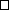that zero value as an assigned cell.
2. For every zero that becomes assigned, cross out (X) all other zeros in the same row and the same column.
3. If for a row and a column, there are two or more zeros and one cannot be chosen by inspection, choose the cell arbitrarily for assignment.
4. The above process may be continued until every zero cell is either assignedor crossed (X).

#### Step 4

An optimal assignment is found, if the number of assigned cells equals the number of rows (and columns). In case you have chosen a zero cell arbitrarily, there may be alternate optimal solutions. If no optimal solution is found, go to step 5.

Table

Use Horizontal Scrollbar to View Full Table Calculation

Job
Person 1 2 3 4
A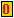5 1 7
B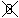3 7 1
C 23 6
D 2#### Step 5

Draw the minimum number of vertical and horizontal lines necessary to cover all the zeros in the reduced matrix obtained from step 3 by adopting the following procedure:

1. Mark all the rows that do not have assignments.
2. Mark all the columns (not already marked) which have zeros in the marked rows.
3. Mark all the rows (not already marked) that have assignments in marked columns.
4. Repeat steps 5 (ii) and (iii) until no more rows or columns can be marked.
5. Draw straight lines through all unmarked rows and marked columns.

You can also draw the minimum number of lines by inspection.

Table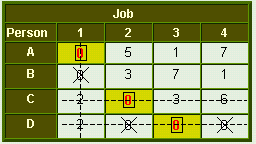#### Step 6

Select the smallest element (i.e., 1) from all the uncovered elements. Subtract this smallest element from all the uncovered elements and add it to the elements, which lie at the intersection of two lines. Thus, we obtain another reduced matrix for fresh assignment.

Table

Job
Person 1 2 3 4
A 0 4 0 6
B 0 2 6 0
C 3 0 3 6
D 3 0 0 0

Now again make the assignments for the reduced matrix.

#### Final Table: Hungarian Method

Job
Person 1 2 3 4
A46
B2 6C 33 6
D 3Since the number of assignments is equal to the number of rows (& columns), this is the optimal solution.

The total cost of assignment = A1 + B4 + C2 + D3

Substituting values from original table:
20 + 17 + 17 + 24 = Rs. 78.

•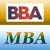MBA & BBA
•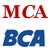BCA & MCA
•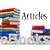Ebooks & Articles
•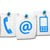Contact us